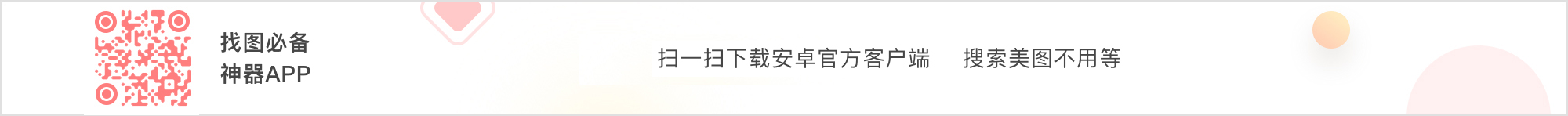# 卖萌

0
3

0
3
♡韩系+女生+欧美+半身+唯美+遮脸+镜子照+中式风+安静+可爱+卖萌+个性+自拍+狗狗+猫猫+森系+背影杀手+侧面+黑白+情头+女生头像+小清新+闺蜜+动漫+双人+四宫格+白边+双马尾+眼妆+自调+手控+腿控+软妹少女心+♡ 图已修
1
3

0
3

0
1
#手帐术# 小编已经教了九种字体了，神马？还不够萌？！说好的会卖萌的大标题来啦！只是几笔就能让你的标题充满感情！不要再说“手残党看看就好”了，小编看烦了！不要再满足于大标题只是把字写大一点好嘛！ ​​​​
0
1
♡韩系+女生+欧美+半身+唯美+遮脸+镜子照+中式风+安静+可爱+卖萌+个性+自拍+狗狗+猫猫+森系+背影杀手+侧面+黑白+情头+女生头像+小清新+闺蜜+动漫+双人+四宫格+白边+双马尾+眼妆+自调+手控+腿控+软妹少女心+♡ 图已修
1
3
♡韩系+女生+欧美+半身+唯美+遮脸+镜子照+中式风+安静+可爱+卖萌+个性+自拍+狗狗+猫猫+森系+背影杀手+侧面+黑白+情头+女生头像+小清新+闺蜜+动漫+双人+四宫格+白边+双马尾+眼妆+自调+手控+腿控+软妹少女心+♡ 图已修
2
5
♡韩系+女生+欧美+半身+唯美+遮脸+镜子照+中式风+安静+可爱+卖萌+个性+自拍+狗狗+猫猫+森系+背影杀手+侧面+黑白+情头+女生头像+小清新+闺蜜+动漫+双人+四宫格+白边+双马尾+眼妆+自调+手控+腿控+软妹少女心+♡ 图已修
1
3

1
3

0
0

0
0

0
0

1
4
♡韩系+女生+欧美+半身+唯美+遮脸+镜子照+中式风+安静+可爱+卖萌+个性+自拍+狗狗+猫猫+森系+背影杀手+侧面+黑白+情头+女生头像+小清新+闺蜜+动漫+双人+四宫格+白边+双马尾+眼妆+自调+手控+腿控+软妹少女心+♡ 图已修
0
12

0
0

0
0

0
0
♡韩系+女生+欧美+半身+唯美+遮脸+镜子照+中式风+安静+可爱+卖萌+个性+自拍+狗狗+猫猫+森系+背影杀手+侧面+黑白+情头+女生头像+小清新+闺蜜+动漫+双人+四宫格+白边+双马尾+眼妆+自调+手控+腿控+软妹少女心+♡ 图已修
0
4
♡韩系+女生+欧美+半身+唯美+遮脸+镜子照+中式风+安静+可爱+卖萌+个性+自拍+狗狗+猫猫+森系+背影杀手+侧面+黑白+情头+女生头像+小清新+闺蜜+动漫+双人+四宫格+白边+双马尾+眼妆+自调+手控+腿控+软妹少女心+♡ 图已修
1
23